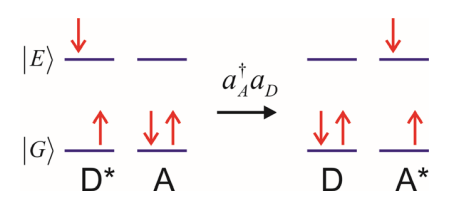# 15.1: Electronic Interactions

$$\newcommand{\vecs}{\overset { \rightharpoonup} {\mathbf{#1}} }$$ $$\newcommand{\vecd}{\overset{-\!-\!\rightharpoonup}{\vphantom{a}\smash {#1}}}$$$$\newcommand{\id}{\mathrm{id}}$$ $$\newcommand{\Span}{\mathrm{span}}$$ $$\newcommand{\kernel}{\mathrm{null}\,}$$ $$\newcommand{\range}{\mathrm{range}\,}$$ $$\newcommand{\RealPart}{\mathrm{Re}}$$ $$\newcommand{\ImaginaryPart}{\mathrm{Im}}$$ $$\newcommand{\Argument}{\mathrm{Arg}}$$ $$\newcommand{\norm}{\| #1 \|}$$ $$\newcommand{\inner}{\langle #1, #2 \rangle}$$ $$\newcommand{\Span}{\mathrm{span}}$$ $$\newcommand{\id}{\mathrm{id}}$$ $$\newcommand{\Span}{\mathrm{span}}$$ $$\newcommand{\kernel}{\mathrm{null}\,}$$ $$\newcommand{\range}{\mathrm{range}\,}$$ $$\newcommand{\RealPart}{\mathrm{Re}}$$ $$\newcommand{\ImaginaryPart}{\mathrm{Im}}$$ $$\newcommand{\Argument}{\mathrm{Arg}}$$ $$\newcommand{\norm}{\| #1 \|}$$ $$\newcommand{\inner}{\langle #1, #2 \rangle}$$ $$\newcommand{\Span}{\mathrm{span}}$$

In this section we will describe processes that result from the interaction between two or more molecular electronic states, such as the transport of electrons or electronic excitation. This problem can be formulated in terms of a familiar Hamiltonian

$H = H _ {0} + V$

in which $$H_o$$ describes the electronic states (including any coupling to nuclear motion), and $$V$$ is the interaction between the electronic states. In formulating such a problem we will need to consider some basic questions: Is V strong or weak? Are the electronic states described in a diabatic or adiabatic basis? How do nuclear degrees of freedom influence the electronic couplings? For weak couplings, we can describe the transport of electrons and electronic excitation with perturbation theory drawing on Fermi’s Golden Rule:

\begin{align} \overline {w} &= \frac {2 \pi} {\hbar} \sum _ {k , \ell} p _ {\ell} \left| V _ {k \ell} \right|^{2} \delta \left( E _ {k} - E _ {\ell} \right) \\[4pt] &= \frac {1} {\hbar^{2}} \int _ {- \infty}^{+ \infty} d t \left\langle V _ {I} (t) V _ {I} ( 0 ) \right\rangle \end{align}

This approach underlies common descriptions of electronic energy transport and non-adiabatic electron transfer. We will discuss this regime concentrating on the influence of vibrational motions they are coupled to. However, the electronic couplings can also be strong, in which case the resulting states become delocalized. We will discuss this limit in the context of excitons that arise in molecular aggregates.

To begin, it is useful to catalog a number of electronic interactions of interest. We can use some schematic diagrams to illustrate them, emphasizing the close relationship between the various transport processes. However, we need to be careful, since these are not meant to imply a mechanism or meaningful information on dynamics. Here are a few commonly described processes involving transfer from a donor molecule D to an acceptor molecule A:

## a) Resonance Energy Transfer

Applies to the transfer of energy from the electronic excited state of a donor to an acceptor molecule. Arises from a Coulomb interaction that is operative at long range, i.e., distances large compared to molecular dimensions. Requires electronic resonance. Named for the first practical derivations of expressions describing this effect: Förster Resonance Energy Transfer (FRET)

## b) Electron Transfer Marcus theory.

Nonadiabatic electron transfer. Requires wavefunction overlap.Ground state Electron TransferExcised state Electron TransferHole Transfer## c) Electron-exchange energy transfer

Dexter Transfer. Requires wavefunction overlap. Singlet or triplet## d) Singlet FissionThis page titled 15.1: Electronic Interactions is shared under a CC BY-NC-SA 4.0 license and was authored, remixed, and/or curated by Andrei Tokmakoff via source content that was edited to the style and standards of the LibreTexts platform; a detailed edit history is available upon request.